Click to Chat

1800-1023-196

+91-120-4616500

CART 0

• 0

MY CART (5)

Use Coupon: CART20 and get 20% off on all online Study Material

ITEM
DETAILS
MRP
DISCOUNT
FINAL PRICE
Total Price: Rs.

There are no items in this cart.
Continue Shopping• Complete Physics Course - Class 11
• OFFERED PRICE: Rs. 2,968
• View Details

Centre of Gravity and Centre of Mass

Table of Content

Centre of Gravity (C.G)

Centre of Mass

The Centre of Mass After Removal of a Part of a Body

Centre of Mass for a Continuous Distribution

Motion of Centre of Mass

Equilibrium

State of Equilibrium

Condition for Stable Equilibrium

Related Resources

Centre of Gravity (C.G)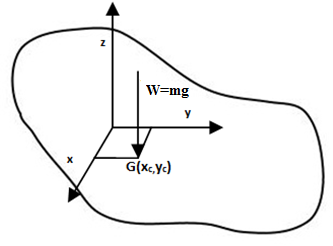Consider a  body of mass m consisting of a number of particles of masses m1, m2,...., mn. Forces m1g, m2g.....mng act on different particles in a direction vertically downward. Thus, the resultant ‘W’ of these parallel forces act at a single point ‘G’ which is called the center of gravity (C.G) of the body.

Center of gravity of a body is a point, through which the resultant of all the forces experienced the various particles of the body, due to attraction of earth, passes irrespective of the orientation of the body.

For regular bodies the position of the center of gravity can be easily located. In case of a thin rod it lies at the center of rod while in case of a rectangular or a square lamina it lies on the point of intersection of their diagonals.

A body when suspended freely by a string must have its center of gravity lying on a vertical line passing through its point of suspension. It is only in that case the condition of equilibrium are fulfilled. So, to locate the C.G. of an irregular body, suspend it by any point and draw a vertical line passing through the point of suspension. Now suspend it form another point and again draw a vertical line passing through the point of suspension. The point of intersection of these two lines gives the center of gravity of the body.

Centre of Mass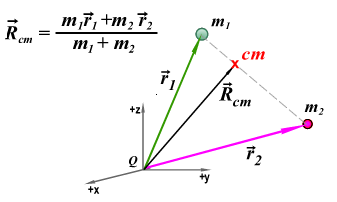Let , , ….. be the position vectors of the different particles of the body with respect to an arbitrary origin Q as shown in the figure.

The center of mass of the system is defined as the point in space, so that moment of the mass of the system about a reference point (origin), when whole of the system is supposed to be concentrated at it (center of mass) is equal to the vector sum of the momenta of the masses, of individual particles around the reference point.

If  is the position vector of the center of mass,

Or,

Here, M = mass of the body.

If the force acting on the body are all in the downward direction center of mass coincides with the center of gravity.

The Centre of Mass After Removal of a Part of a Body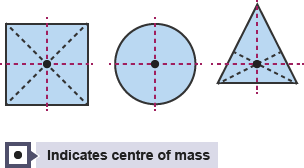If a portion of a body is taken out, the remaining portion may be considered as, original mass (M) -mass of the removed part (m)

= {Original mass (M)} + {- mass of the removed part (m)}

The formula changes to:

Xcm = (Mx-mx')/(M-m) and Ycm = (My-my')/(M-m)

where primed ones represent the coordinate of the C.M. of the removed part.

Refer this simulation for centre of mass

[Note: Please click on “red circle” to run the simulation]

Centre of Mass for a Continuous Distribution

For a continuous distribution of mass, we can treat an element of mass dm at any position as a point mass and replace the summation by integration as shown below:

Rcm = 1/M ∫ x dm

So, we get Xcm = 1/M ∫ x dm

Ycm = 1/M ∫ y dm

Zcm = 1/M ∫ z dm,

Note:For centre of mass the following are self-explanatory.(1) There may or may not be any mass present at the centre of mass as can be seen in the figures below. In the first body there is mass present at the centre of mass but in the second example of a ring there is no mass at the centre of mass.

(2) Its position depends on the shape of the body. It is nearer to the region where more mass is concentrated (because of vector r).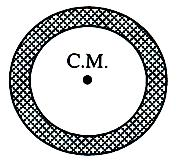(3) For symmetrical bodies having homogeneous distribution of mass coincides with the centre of symmetry (again think in terms of vector r).

(4) Centre of mass and centre of gravity need not be at the same points (consider a very high mountain and apply the concept of variation of g with height).

Motion of Centre of Mass

Now that we have the position, we extend the concept of the center of mass to velocity and acceleration, and thus give ourselves the tools to describe the motion of a system of particles. Taking a simple time derivative of our expression for x cm we see that:

Vcm = m1v1+m2v2/m1+m2

Thus we have a very similar expression for the velocity of the center of mass. Differentiating again, we can generate an expression for acceleration:

acm = m1a1+m2a2/m1+m2

With this set of three equations we have generated the necessary elements of the kinematics of a system of particles.

So, (m1+m2) acm =  m1a1+m2a2

Fcm = F1+ F2 + ….

Here, Fcm = M (acm)

Here M is the total mass of the system.

Hence the total mass of the system times the acceleration of its centre of mass is equal to vector sum of all the forces acting on the group of particles.

Refer this video to know more about on centre of mass

Equilibrium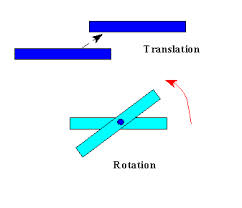A rigid body is said to be in equilibrium if the forces acting on it do not change its state of rest or of uniform motion (linear or rotational).

This means if a body is at rest it should remain at rest. If the body is in motion, it should keep on moving with uniform velocity (may be linear or angular). Accordingly the equilibrium is classified into following two categories:

(a) Translatory equilibrium

A body is said to be in translatory equilibrium if its centre of mass possess no linear acceleration in an inertial frame of reference. For a body to be in translatory equilibrium the basic condition is that the vector sum of all the external forces acting on the body should be zero. In such case a body at rest will remain at rest. This equilibrium is static equilibrium. A body moving with uniform velocity, along a straight line, will keep on doing so. This equilibrium is termed as dynamic translatory equilibrium.

(b) Rotatory equilibrium

A body is said to be in rotatory equilibrium if it possesses no angular acceleration about any axis in an inertial frame.

For the body to be in rotatory equilibrium the basic condition is that the vector sum of all the external torques acting on the body is zero.

For a body to be in equilibrium is must satisfy both the conditions stated above simultaneously, i.e.,

(a) the vector sum of all the external forces acting on the body should vanish.

(b) the vector sum of all the external torque acting on the body should vanish.

State of Equilibrium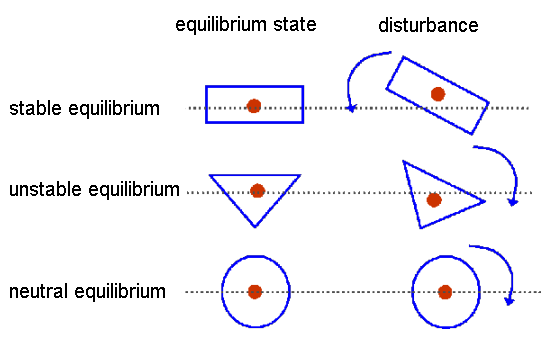Equilibrium can be classified into three categories

(a) Stable equilibrium

The equilibrium of a body is said to stable if, on being slightly disturbed, it tends to come back to its original position.

(b) Unstable equilibrium

The body is said to be in unstable equilibrium if on being slightly disturbed, it shows no tendency to come back to its original position and moves away from it.

(c) Neutral equilibrium

A body is said to be in neutral equilibrium if on being slightly displaced, it remains in the new position.

Condition for Stable Equilibrium

The degree of stability of the depends upon the height of the centre of gravity of the body from the surface of support. Smaller the height of centre of gravity, greater is its stability.

(a) The vertical drawn from center of gravity should pass through the base.

(b) The center of gravity should be as low as possible.

(c) The base should be as wide as possible.The center of mass is the unique point at the center of a distribution of mass in space that has the property that the weighted position vectors relative to this point sum to zero.

The center of mass is the location where all of the mass of the system could be considered to be located.

For a solid body it is often possible to replace the entire mass of the body with a point mass equal to that of the body's mass. This point mass is located at the center of mass.

For homogenous solid bodies that have a symmetrical shape, the center of mass is at the center of body's symmetry, its geometrical center.

The center of mass is the point about which a solid will freely rotate if it is not constrained.

For a solid body the center of mass is also the balance point. The body could be suspended from its center of mass and it would not rotate, i.e. not be out of balance.

The center of mass of a solid body does not have to lie within the body. The center of mass of a hula-hoop is at its center where there is no hoop, just hula.

The center of mass for a system of independently moving particles still has meaning and is useful in analyzing the interactions between the particles in the system.

if the object is symmetric and the mass is distributed uniformly throughout the object's volume, then the center of mass lies on an axis of symmetry

If an object is pivoted on its center of gravity it balances in any orientation (no rotation).

If the acceleration of gravity  is the same throughout the object, then the center of gravity coincides with the center of mass.

The center of mass (COM) is the unique point at the center of a distribution of mass in space that has the property that the weighted position vectors relative to this point sum to zero.

An object thrown through the air may spin and rotate, but its center of gravity will follow a smooth parabolic path, just like a ball.

If you tilt an object, it will fall over only when the center of gravity lies outside the supporting base of the object.

If you suspend an object so that its center of gravity lies below the point of suspension, it will be stable. It may oscillate, but it won't fall over.

The center of mass of an object of uniform density is the body’s geometric center. Note that the center of mass does not need to be located within the object itself.

A particle of mass 1 kg is projected upwards with velocity 60 m/s. Another particle of mass 2 kg is just dropped from a certain height. After 2s when neither of the particles have collided with ground. Find out acceleration and velocity of COM.

Solution:

We know that, aCOM = [m1a1 + m2a2]/[m1+m2] and uCOM = [m1u1 + m2u2]/[m1+m2]

So, aCOM = [m1a1 + m2a2]/[m1+m2]

= [(1) (-10) + 2 (-10)] / 3

= -10 m/s2

uCOM = m1u1 + m2u2/m1+m2

= [(1) (60) + (2) (0)]/3 = + 20 m/s

From the above observation we conclude that, acceleration of the centre of mass will be -10 m/s2 nad velocity of the centre of mass will be 20 m/s.

Problem:

A system consisting of two objects has a total momentum of (18 kgm/sec)i and its center of mass has the velocity of (3 m/s)i.One of the object has the mass 4 kg and velocity (1.5 m/s)i.The mass and velocity of the other

objects are

(a) 2 kg, (6 m/s)i          (b) 2 kg, (-6 m/s)i

(c)2 kg, (3 m/s)i           (d) 2 kg, (-3 m/s)i

Solution:

Given, Total momentum=(18 kgm/sec)i, velocity of Center of mass=(3 m/s)i, Mass of one object=4 kg, Velocity of this object=(1.5 m/s)i

Let m be the mass of other object and v be the velocity.

Now we know total momentum =Total massX velocity of center of mass

(18 kgm/sec)i=(m+4)(3 m/s)i.

or m=2 kg

Now Vcm=(m1v1+m2v2)/(m1+m2)

Or, 3i=(41.5i + 2v)/6

So, 18i=6i+2v

Or, v=6i m/sec

From the above observation, we conclude that option (a) is correct.

Problem:Particle of masses 2 kg, 2 kg, 1 kg and 1 kg are placed at the corners A, B, C, D of a square of side L as shown in the figure. Find the centre of mass of the system.

Solution:

If A is taken as origin, then,

xcm = (m1 x1 + m2 x2 + m3 x3 + m4 x4) / (m1 + m2 + m3 + m4 )

= (2.0+2.L+1.L+1.0)/6 = 1/2

ycm = (m1 x1 + m2 x2 + m3 x3 + m4 x4) / (m1 + m2 + m3 + m4)

= (2.0+2.0+1.L+1.L)/6=L/3

Problem: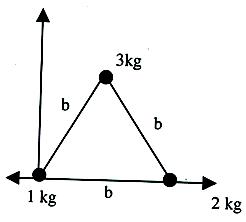Locate the centre of mass of the given system of three particles located at the vertices of an equilateral triangle.

Solution:

xcm = (1×0+2×0+3(b/2))/(1+2+3) = 7/12

ycm = (1×0+2×0+3√3(b/2))/(1+2+3) = (3b√3)/12.

Problem:

A dog of mass 10 kg is standing on a flat 10 m long boat so that is 20 meters from the shore. It walks 8 m on the boat towards the shore and then stops. The mass of the boat is 40 kg and friction between the boat and th

water surface is negligible. How far is the dog from the shore now?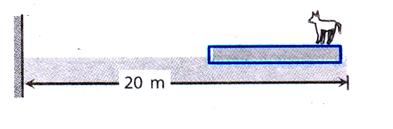Solution:

Take boat and dog as a system. Initially, centre of mass of the system is at rest. Since no external force is acting on the system, hence centre of mass

of the system will remain stationary. Let initially distance of the centre of mass of the boat from the shore be x m.

Then,   x1.c.m. = (40 × x × 10 × 20)/(40 + 10) m …… (i)

Here,  x1.c.m. = distance of the C.M. of the system from the shore.

Since dog moves towards the shore, for the centre of mass of the system to be at rest, the boat has to move away from the shore. Let distance moved by the boat be ‘x’. Then,

x2.c.m. = (40 (x+x' ) + 10(20 - 8 + x'))/(40 + 10)

As x1.c.m. = x2.c.m.

=> (40x + 200)/50 + (40(x + x' ) + 10(12 + x'))/50

=> 50x’ = 80 => x’ = 1.6 m.

Hence, distance of the dog from the shore is (20 – 8 + 1.6)m = 13.6 cmQuestion 1

A cricket bat is cut at the location of its centre of mass as shown. Then,

(a) the two pieces will have the same mass

(b) the bottom piece will have larger mass

(c) the handle piece will have larger mass

(d) mass of handle piece is double the mass of bottom piece

Question 2

The center of mass of a rigid body :

(a) coincides with geometric center.

(b) is a geometric point.

(c) lies always inside the rigid body.

(d) lies always outside the rigid body.

Question 3

The density of a rod is not constant. In which of the following situation COM cannot lie at the geometric center ?

(a) Density increases from left to right for the first half and decreases from right to left for the second half

(b) Density increases from left to right

(c) Density decreases from left to right

(d) Density decreases from left to right for the first half and increases from right to left for the second half

Question 4

The center of gravity is usually located where

?(a) more weight is concentrated

?(b) less weight is concentrated

?(c) less mass is concentrated

?(d) more mass is concentrated

Question 5

The stationary sail boat air is blown at the sails from a fan attached to the boat. The boat will :

(a) move in the direction opposite to that in which air is blown

(b) move in the direction in which air blown

(c) spin around

(d) remain at restQ.1
Q.2
Q.3
Q.4
Q.5

b

b

b and c

d

c

Related Resources

You might like to Projectile Motion.

For getting an idea of the type of questions asked, refer the  Previous Year Question Papers.

To get answer to any question related to centre of gravity and centre of mass click here.### Course Features

• 101 Video Lectures
• Revision Notes
• Previous Year Papers
• Mind Map
• Study Planner
• NCERT Solutions
• Discussion Forum
• Test paper with Video Solution